Checkout JEE MAINS 2022 Question Paper Analysis : Checkout JEE MAINS 2022 Question Paper Analysis :

# Parallel Plate Capacitor

## What is a Parallel Plate Capacitor?

Parallel Plate Capacitors are formed by an arrangement of electrodes and insulating material or dielectric. A parallel plate capacitor can only store a finite amount of energy before dielectric breakdown occurs. It can be defined as:

When two parallel plates are connected across a battery, the plates are charged and an electric field is established between them, and this setup is known as the parallel plate capacitor.

Understand the working principle of a parallel plate capacitor clearly by watching the video.## Parallel Plate Capacitor Formula

The direction of the electric field is defined as the direction in which the positive test charge would flow. Capacitance is the limitation of the body to store the electric charge. Every capacitor has its capacitance. The typical parallel-plate capacitor consists of two metallic plates of area A, separated by the distance d.

The parallel plate capacitor formula is given by:

 $$\begin{array}{l}C=k\epsilon _{0}\frac{A}{d}\end{array}$$

Where,

• ϵo is the permittivity of space (8.854 × 10−12 F/m)
• k is the relative permittivity of dielectric material
• d is the separation between the plates
• A is the area of plates

## Parallel Plate Capacitor Derivation

The figure below depicts a parallel plate capacitor. We can see two large plates placed parallel to each other at a small distance d. The distance between the plates is filled with a dielectric medium as shown by the dotted array. The two plates carry an equal and opposite charge.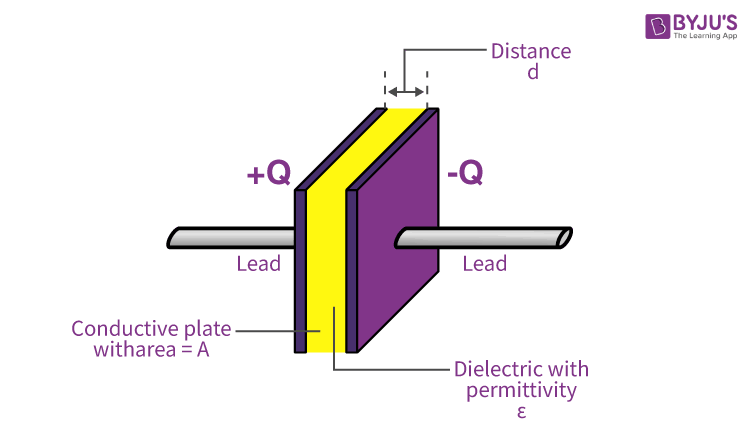Parallel Plate Capacitor

Here, we see that the first plate carries a charge +Q and the second carries a charge –Q. The area of each of the plates is A and the distance between these two plates is d. The distance d is much smaller than the area of the plates and we can write d<<A, thus the effect of the plates are considered as infinite plane sheets and the electric field generated by them is treated as that equal to the electric field generated by an infinite plane sheet of uniform surface charge density. As the total charge on plate 1 is Q and the area of the plate is A, the surface charge density can be given as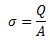Similarly, for plate 2 with a total charge equal to –Q and area A, the surface charge density can be given as,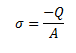We divide the regions around the parallel plate capacitor into three parts, with area 1 being the area left to the first plate, area 2 being the area between the two planes and area 3 is the area to the right of plate 2.

Let us calculate the electric field in the region around a parallel plate capacitor.

Region I: The magnitude of the electric field due to both the infinite plane sheets I and II is the same at any point in this region, but the direction is opposite to each other, the two forces cancel each other and the overall electric field can be given as,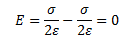Region II: The magnitude, as well as the direction of the electric field due to both the plane sheets I and II in these regions, is the same and the overall effect can be given as,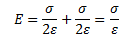Region III:  Similar to the region I, here too, the magnitude of the electric field generated due to both the plane sheets I and II is the same but the direction is opposite, giving the same result as,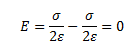Here, the electric field is uniform throughout and its direction is from the positive plate to the negative plate.

The potential difference across the capacitor can be calculated by multiplying the electric field and the distance between the planes, given as,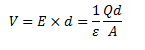And the capacitance for the parallel plate capacitor can be given as,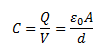You may also want to check out these topics given below!

## Solved Examples

### Example 1

A parallel plate capacitor is kept in the air has an area of 0.50m2 and separated from each other by distance 0.04m. Calculate the parallel plate capacitor.

Solution:

Given:

Area A = 0.50 m2,

Distance d = 0.04 m,

relative permittivity k = 1,

ϵo = 8.854 × 10−12 F/m

The parallel plate capacitor formula is expressed by,

$$\begin{array}{l}C=k\frac{\epsilon _{0}A}{d}\end{array}$$

= 8.854×10−12 × 0.50 / 0.04

= 4.427 x 10−12 / 0.04

Therefore, C = 110.67 x 10−12 F

### Example 2

Determine the area of parallel plate capacitor in the air if the capacitance is 25 nF and separation between the plates is 0.04m.

Solution:

Given:

Capacitance = 25 nF,

Distance d = 0.04 m,

Relative permittivity k = 1,

ϵ= 8.854 × 10−12 F/m

The parallel plate capacitor formula is expressed by,

$$\begin{array}{l}C=k\frac{\epsilon _{0}A}{d}\end{array}$$
$$\begin{array}{l}A=\frac{dC}{k\epsilon _{0}}\end{array}$$

= 0.04 × 25×10−9 / 1×8.854×10−12

A = 1 x10−9 / 8.854 ×10−12

Therefore, area of parallel plate capacitor is 112.94 m2.

To know more, visit BYJU’S – The learning app!

## Frequently Asked Questions – FAQs

### What are capacitors?

Capacitors are electronic devices that store electrical energy in an electric field. They are passive electronic components with two distinct terminals.

### What is meant by capacitance?

Capacitance is the amount of electric charge that can be stored per unit change in electric potential.

### What are parallel plate capacitors?

When two parallel plates are attached over a battery, the given plates are gradually charged, and an electric field is produced between them. These setups are called the parallel plate capacitors.